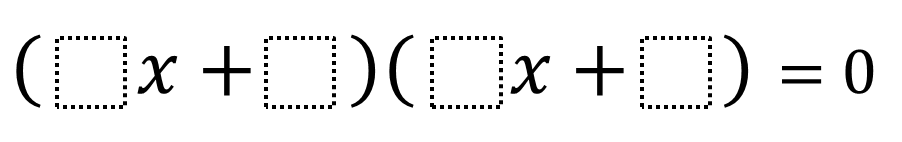Directions: Use only the digits 1-9, each digit only once, create a problem that has the solutions x = 4 and x = -1/2.### Hint

If you were to solve this equation, what would your first step be?

How does the Zero Product Property help us rewrite this equation?

What would the parameters need to be in the first factor to result in a solution of x = 4?

If (ax + b)(cx + d) = 0, there are infinite answers of the form -b/a = 4 and -d/c = -1/2.

Most commonly found student answer: (1x + -4)(2x + 1) = 0.

Source: Amy Herzog

## Difference of Squares and Sum of Cubes

Directions: Using the digits 0 to 9 at most one time each, place a digit …

1.••